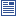Accessibility options:

# Second order differential equations resources

Show me all resources applicable to

### Community Project (1)Second Order Ordinary Differential Equations (SOURCE)
A zip file containing the LaTeX source files and metadata for the Teach Yourself leaflet on Second Order Differential Equations: A summary of how to solve second order ODEs with constant coefficients: how to solve homogeneous equations (with no right hand-side) and how to solve equations with a right hand-side (method of undetermined coefficients and methods of variation of parameters). This Teach Yourself resource is contributed to the mathcentre Community Project by Morgiane Richard, University of Aberdeen and is reviewed by Shazia Ahmed, University of Glasgow.

### Teach Yourself (1)Second Order Ordinary Differential Equations
Second Order Differential Equations: A summary of how to solve second order ODEs with constant coefficients: how to solve homogeneous equations (with no right hand-side) and how to solve equations with a right hand-side (method of undetermined coefficients and methods of variation of parameters). This Teach Yourself resource is contributed to the mathcentre Community Project by Morgiane Richard, University of Aberdeen and is reviewed by Shazia Ahmed, University of Glasgow.

### Test Yourself (2)Differential Equations Test 01 (DEWIS)
Four questions on second order linear constant coefficient differential equations. The first two involve identifying the complementary function, the third involves applying initial conditions and the fourth involves finding a particular solution with either linear or sinusoidal forcing. DEWIS resources have been made available under a Creative Commons licence by Rhys Gwynllyw & Karen Henderson, University of the West of England, Bristol.Second order differential equations - Numbas
5 questions on second order differential equations. First two and last, linear with constant coefficients; first two homogeneous, complex and repeated roots. Last, non-homogeneous. Third, motion under gravity. Fourth, linear with a given particular solution (variation of parameters). Numbas resources have been made available under a Creative Commons licence by the School of Mathematics & Statistics at Newcastle University.